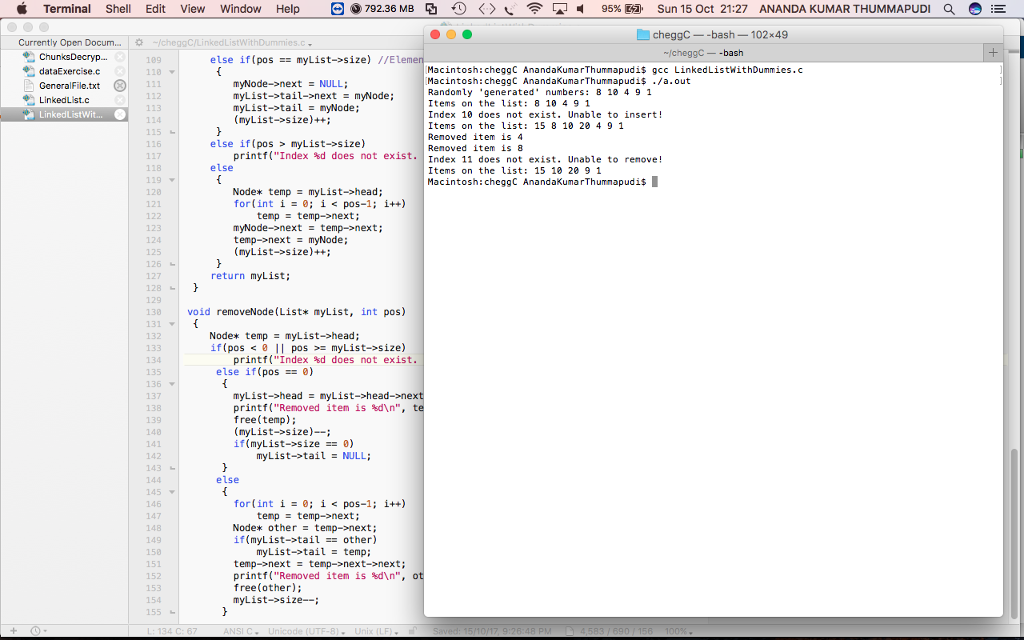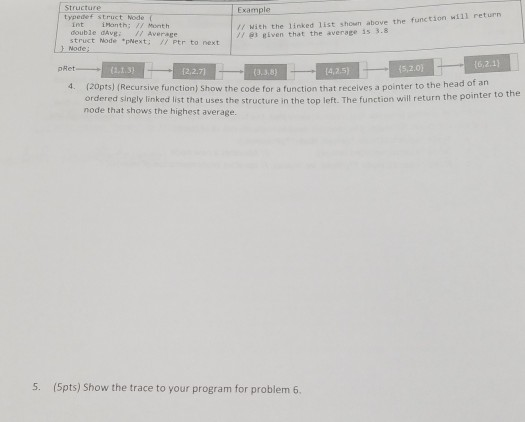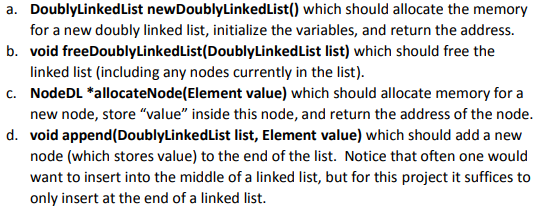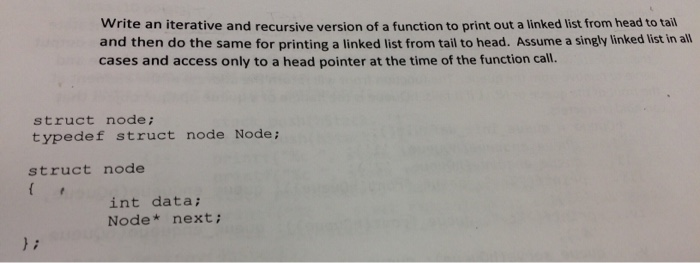# IN C Programming #include<stdio.h> #include<stdlib.h> typedef struct nodestruct { int item; struct nodestruct *next; } Node;...

IN C Programming

#include<stdio.h>

#include<stdlib.h>

typedef struct nodestruct {

int item;

struct nodestruct *next;

} Node;

typedef struct {

int size; // Number of items on user’s list

} List;

//In addition to creating an empty list, this function will also create two dummy nodes; one for the head, and the other for the tail.
List* createList();

//This function creates a node containing the provided item, then inserts it into the list pointed by the provided list pointer at a location provided by the index. Finally, it should return an updated list pointer. Note: index is zero based, starting from the first node following the dummy node. The function should be able to print out an error message if the index is beyond the size of the list and returns the un-updated list pointer.
List* insertNode(List* , int, int);

//This function removes the node at the location provided by the index from the provided list pointer. Note: index is zero based, starting from the first node following the dummy node. The function should be able to print out an error message if the index is beyond the size of the list.
void removeNode(List*, int);

Hint: Modify the printList function since we use dummy nodes here.

Main description:
1. Using the provided two ADTs, create a list with two dummy nodes.
2. Generate an array with 5 random integer numbers between 1 and 10. Print out the 'generated' numbers on the console separated by space.
3. Insert each of the generated numbers in the list using the example FOR LOOP as below.
for (int i = 0; i<5; i++){
list = insertNode(list, i, array[i]);
}
4. Print the contents of the list on the console separated by space.
5. Now, insert 15 at the 0th index of the list. Next, insert 20 at the 3rd index of the list. Finally insert 25 at the 10th index of the list.
6. Print the contents of the list on the console separated by space.
7. Remove the node at the 4th index of the list. Next, remove the node at the 1st index of the list. Finally remove the 11th index of the list.
8. Print the contents of the list on the console separated by space.

Example output:

mwc-070138:~ \$ gcc main.c lab8.c -Wall -Werror
mwc-070138:~ \$ ./a.out
Randomly 'generated' numbers: 1 3 7 2 10

Items on the list: 1 3 7 2 10

Index 10 does not exist. Unable to insert!

Items on the list: 15 1 3 20 7 2 10

Removed item is 7
Removed item is 1
Index 11 does not exist. Unable to remove!

Items on the list: 15 3 20 2 10

Here is the code for you:

#include<stdio.h>
#include<stdlib.h>

typedef struct nodestruct {
int item;
struct nodestruct *next;
} Node;

typedef struct {
int size; // Number of items on user’s list
} List;

//In addition to creating an empty list, this function will also create two dummy nodes;
//one for the head, and the other for the tail.
List* createList();

//This function creates a node containing the provided item, then inserts it into the list
//pointed by the provided list pointer at a location provided by the index. Finally, it
//should return an updated list pointer. Note: index is zero based, starting from the
//first node following the dummy node. The function should be able to print out an error
//message if the index is beyond the size of the list and returns the un-updated list pointer.
List* insertNode(List* , int, int);

//This function removes the node at the location provided by the index from the provided
//list pointer. Note: index is zero based, starting from the first node following the
//dummy node. The function should be able to print out an error message if the index is
//beyond the size of the list.
void removeNode(List*, int);

//Hint: Modify the printList function since we use dummy nodes here.
void printList(List*);

int main()
{
//Main description:
//1. Using the provided two ADTs, create a list with two dummy nodes.
List *myList = createList();

//2. Generate an array with 5 random integer numbers between 1 and 10.
//Print out the 'generated' numbers on the console separated by space.
int array;
for(int i = 0; i < 5; i++)
array[i] = rand() % 10 + 1;
printf("Randomly 'generated' numbers: ");
for(int i = 0; i < 5; i++)
printf("%i ", array[i]);
printf("\n");

//3. Insert each of the generated numbers in the list using the example FOR LOOP as below.
for (int i = 0; i<5; i++){
myList = insertNode(myList, i, array[i]);
}

//4. Print the contents of the list on the console separated by space.
printList(myList);

//5. Now, insert 15 at the 0th index of the list.
//Next, insert 20 at the 3rd index of the list.
//Finally insert 25 at the 10th index of the list.
myList = insertNode(myList, 0, 15);
myList = insertNode(myList, 3, 20);
myList = insertNode(myList, 10, 25);

//6. Print the contents of the list on the console separated by space.
printList(myList);

//7. Remove the node at the 4th index of the list.
//Next, remove the node at the 1st index of the list.
//Finally remove the 11th index of the list.
removeNode(myList, 4);
removeNode(myList, 1);
removeNode(myList, 11);

//8. Print the contents of the list on the console separated by space.
printList(myList);
}
void printList(List* myList)
{
printf("Items on the list: ");
while(temp != NULL)
{
printf("%i ", temp->item);
temp = temp->next;
}
printf("\n");
}
List* createList()
{
List* myList;
myList->size = 0;
return myList;
}

List* insertNode(List* myList, int pos, int value)
{
Node* myNode = malloc(sizeof(Node));
myNode->item = value;
if(pos == 0)   //Element being inserted at the start of the list.
{
if(myList->size == 0)
myList->tail = myNode;
(myList->size)++;
}
else if(pos == myList->size) //Element being inserted at the end of the list.
{
myNode->next = NULL;
myList->tail->next = myNode;
myList->tail = myNode;
(myList->size)++;
}
else if(pos > myList->size)
printf("Index %d does not exist. Unable to insert!\n", pos);
else
{
for(int i = 0; i < pos-1; i++)
temp = temp->next;
myNode->next = temp->next;
temp->next = myNode;
(myList->size)++;
}
return myList;
}

void removeNode(List* myList, int pos)
{
if(pos < 0 || pos >= myList->size)
printf("Index %d does not exist. Unable to remove!\n", pos);
else if(pos == 0)
{
printf("Removed item is %d\n", temp->item);
free(temp);
(myList->size)--;
if(myList->size == 0)
myList->tail = NULL;
}
else
{
for(int i = 0; i < pos-1; i++)
temp = temp->next;
Node* other = temp->next;
if(myList->tail == other)
myList->tail = temp;
temp->next = temp->next->next;
printf("Removed item is %d\n", other->item);
free(other);
myList->size--;
}
}

And the output screenshot is:#### Earn Coin

Coins can be redeemed for fabulous gifts.

Similar Homework Help Questions
• ### Consider a Linked List program with the following class: typedef int datatype; struct node {   datatype...

Consider a Linked List program with the following class: typedef int datatype; struct node {   datatype data; node *tail; }; class LinkedList{ private: node *head; node *current;public: //constructors LinkedList(); LinkedList(int i); //destructor ~LinkedList(); bool start(); //sets list postion to header bool nextNode(); //increments to next node in list int getCurrent(); //returns data from current node void insertNode(int i); //inserts node after current node    //then sets current node to new node bool deleteNode();//deletes currentnode void deleteAll(); //deletes all nodes };...

• ### C PROGRAMMING #include <stdio.h> #include <stdlib.h> struct nodet { int data; struct nodet *link; }; struct...

C PROGRAMMING #include <stdio.h> #include <stdlib.h> struct nodet { int data; struct nodet *link; }; struct nodet *makeAnode(int val) { struct nodet *box; box = malloc(sizeof(struct nodet) ); box->data = val; box->link = NULL; return box; } void printList(struct nodet *L) { struct nodet = *mov; mov = L; while(mov != NULL) { printf("%d ", mov->data); mov = mov->link; } printf("\n"); } // THIS SHOULD COUNT HOW MANY ITEMS (NODES) ARE IN THE LIST. int listLen(struct nodet **L) { int...

• ### CODE IN C Objectives: Queue operations. Data structure: typedef struct { int width; int heig...

CODE IN C Objectives: Queue operations. Data structure: typedef struct { int width; int height; }Rect; typedef struct node { Rect* r; struct node* next; }Node; typedef struct { Node* head; Node* tail; }Queue; Specification: In this lab six Queue-related operation functions need to be implemented by using the given function prototypes and data structures. 1. List* createQueue(void); This function initializes an empty “Queue” with the “Queue” data structure and returns an empty queue. 2. int enQueue(List*); This function receives...

• ### Deleting multiples of a given integer from a linked list: #include <stdio.h> #include <stdlib.h> #include <assert.h>...

Deleting multiples of a given integer from a linked list: #include <stdio.h> #include <stdlib.h> #include <assert.h> #define MAX 10000 typedef struct node_tag { int v; // data struct node_tag * next; // A pointer to this type of struct } node; // Define a type. Easier to use. node * create_node(int v) { node * p = malloc(sizeof(node)); // Allocate memory assert(p != NULL); // you can be nicer // Set the value in the node. p->v = v; p->next...

• ### Given the following linked list structure called node: struct node { int val; struct node *...

Given the following linked list structure called node: struct node { int val; struct node * ptrNext; }; Assume we have a single list created from this structure with a head pointer called ptrFirst which is declared in the global scope. a. Write a complete C function called CountEven to count all the even values in this singly linked list of arbitrary number of nodes using an iterative (non-recursive) approach. The function takes as parameter the pointer to the starting...

• ### Structure struct Node int Manth; // Mont h double dAvg: 1/ Average struct Node pNext // with, the linked İist 3hown above the function will return gven that the average is 3.8 Ptr to next -Nod...Structure struct Node int Manth; // Mont h double dAvg: 1/ Average struct Node pNext // with, the linked İist 3hown above the function will return gven that the average is 3.8 Ptr to next -Node; Ret (3,3.8) (4,2.5) (20pts)( Recursive function) Show the code for a function that receives a pointer to the head of an ordered singly linked list that uses the structure in the top left. The function will return the pointer node that shows the highest...

• ### Create Functions for the following prototypes. Below is the setup.*program is in c* #include <stdio.h> #include...Create Functions for the following prototypes. Below is the setup.*program is in c* #include <stdio.h> #include <string.h> #include <stdarg.h> #include <stdlib.h> //#define constant values #define MAX_URL_LENGTH 50 #define TRUE 1 #define FALSE 0 //typedef for the Element struct which constains a c string to store a URL in the BrowserList typedef struct { char szURL[MAX_URL_LENGTH]; } Element; //Typedef for a node in the doubly linked list (has next and previous pointers). typedef struct NodeDL { Element element; struct NodeDL *pNext;...

• ### C programming Write an iterative and recursive version of a function to print out a linked...C programming Write an iterative and recursive version of a function to print out a linked list from head to tail and then do the same for printing a linked list from tail to head. Assume a singly linked list in all cases and access only to a head pointer at the time of the function call. struct node; typedef struct node Node; struct node int data; Node next;

• ### Code in C language ADT: typedef struct{ int ID; float salary; int age; }Employee; ...

code in C language ADT: typedef struct{ int ID; float salary; int age; }Employee; Specification: In this lab, five functions need to be implemented using the given ADT. 1. Employee* readRecord(FILE*) This function receives a FILE pointer created before. It reads a line from the provided csv file, and creates an Employee struct pointer with the information from that line then returns the pointer back to the calling function. Each line in the provided csv file contains the id, salary,...

• ### In C++ Assuming: struct node { int data; node * next; }; and copyList(node * head);...

In C++ Assuming: struct node { int data; node * next; }; and copyList(node * head); Write a recursive function (copyList(node * head)) that will create a copy of the singly linked list.